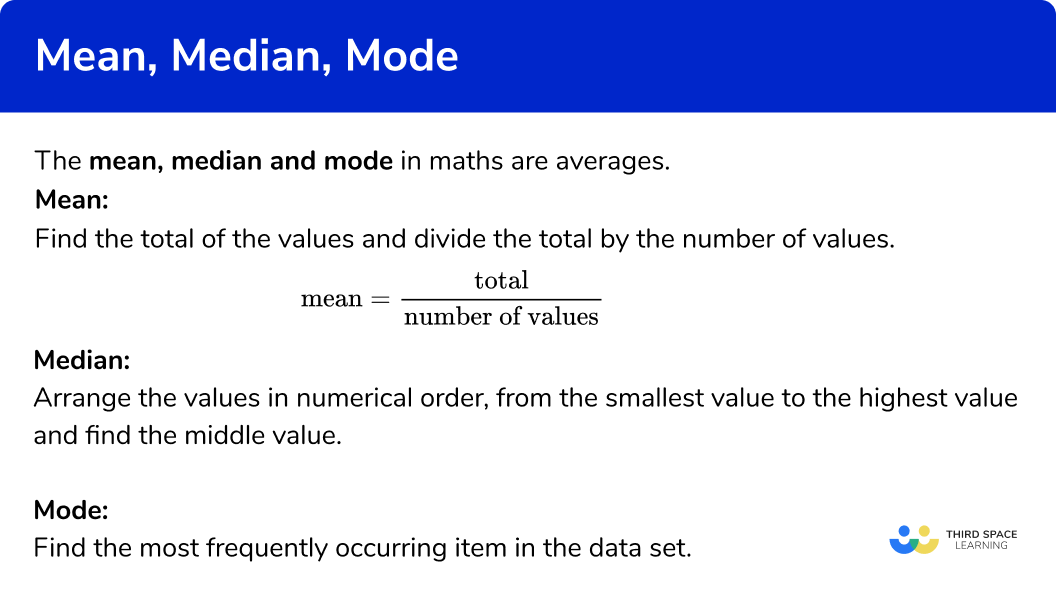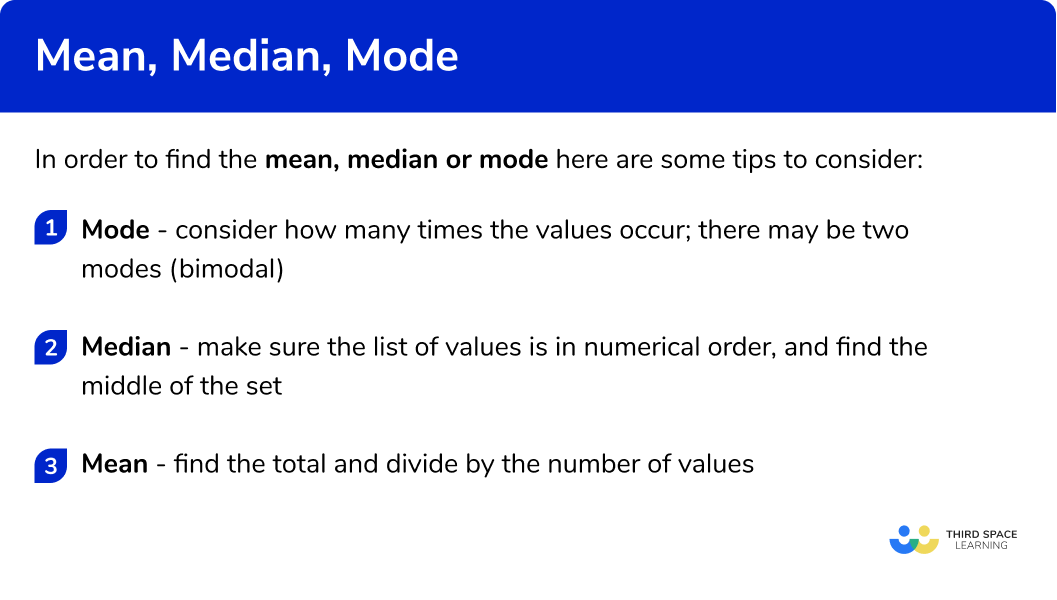GCSE Maths Statistics

Mean, median, mode

# Mean, Median, Mode

Here we will learn about the mean, median and mode, including what they are and how to find them.

There are also mean median mode worksheets based on Edexcel, AQA and OCR exam questions, along with further guidance on where to go next if you’re still stuck.

## What is mean median mode?

The mean, median and mode are averages.

Averages are measures of central tendency, they are values that can be used to represent a set of data.

We will look at three averages: mean, median and mode.

### What is mean median mode?## Mean

To calculate the mean we find the total of the values and divide the total by the number of values.

The “number of values” is sometimes referred to as the “number of numbers”.

\text{Mean}=\frac{\text{total}}{\text{number of values}}

E.g. Find the mean of this set of numbers

\text{Mean}=\frac{\text{total}}{\text{number of values}}=\frac{1+3+4+5+6+6+7}{7}=\frac{32}{7}=4.57 \ \text{(to 2 dp)}

The mean is 4.57 (to 2 dp ).

Step-by-step guide: Mean

## Median

The median is the middle number.

To find the median we need to arrange the values in numerical order, from the smallest value to the highest value and find the middle value.

The middle value is the median value.

E.g. Find the median of this set of numbers

The median is 5 .

Step-by-step guide: Median

## Mode

The mode is the most common number.

To find the mode we need to find the most frequently occurring item in the data set.

E.g. Find the mode of this list of numbers

The most frequently occurring item is ‘6’

The mode is 6 .

Step-by-step guide: Mode or modal

## How to find the mean median mode

In order to find the mean, median or mode:

1. Mode – consider how many times the values occur; there may be two modes (bimodal).
2. Median – make sure the list of values is in numerical order, and find the middle of the set.
3. Mean – find the total and divide by the number of values.

### How to find the mean median mode## Mean median mode examples

### Example 1: finding the mode

Find the mode:

Consider the number of times the different items occur.

7 occurs twice, more than any other item.

The mode is 7

### Example 2: finding the mode

Find the mode:

Consider the number of times the different items occur.

3 and 6 both occur twice so there are two modes. The data is bimodal.

The mode is 3 and 6

### Example 3: finding the median

Find the median:

Make sure the list of numbers is in numerical order.

Find the middle of the data set.

The median is 9

### Example 4: finding the median

Find the median:

Make sure the list of numbers is in numerical order.

Find the middle of the data set. There is an even number of values, so we have a middle pair. The average of 4 and 6 is 5 . (Or the midpoint of 4 and 6 is 5 ).

\frac{4+6}{2}=\frac{10}{2}=5

The median is 5

### Example 5: finding the mean

Find the mean:

Find the total and divide by the number of values

\text{Mean}=\frac{\text{total}}{\text{number of values}}=\frac{7+8+6+8+9}{5}=\frac{38}{5}=7.6

The mean is 7.6

### Example 6: finding the mean

Find the mean:

Find the total and divide by the number of values

\text{Mean}=\frac{\text{total}}{\text{number of values}}=\frac{11+13+16+12+12+14}{6}=\frac{78}{6}=13

The mean is 13

### Common misconceptions

• Mixing up the averages

Check if you have been asked for the median, mode or mean average.

• No mode?

A data set can have 1 mode or 2 modes (bimodal) etc. But it is possible that a data set has no mode at all.

• Ascending order

Check that the list of numbers is in numerical order before finding the median number.

• Decimal averages

The mean does not have to be a whole number. It can be a decimal or a fraction. It may be a decimal which needs rounding.

### Practice mean median mode questions

1. Find the mode:12171816The most common item is ‘18’ . The number of times ‘18’ occurs is 2 times. The other numbers only occur once.

2. Find the mode:19212319 and 23The most common items are ‘19’ and ‘23’ . These two items occur twice. The other numbers only occur once.

3. Find the median:18191731Put the numbers in order from the smallest to largest value. Then find the middle value.The middle value is 17 , so this is the median.

4. Find the median:35363738Put the numbers in order from the smallest to largest value. Then find the middle value.The middle pairs of values are 35 and 37 . The average of these is 36 . The median is 36 .

\frac{35+37}{2}=\frac{72}{2}=36

5. Find the mean:1712.516.415.5\text{Mean}= \frac{\text{total}}{\text{number of values}} \text{Mean}=\frac{18+12+15+17}{4} \text{Mean}= \frac{62}{4} \text{Mean}=15.5

6. Find the mean:6875\text{Mean}= \frac{\text{total}}{\text{number of values}}\\ \text{Mean}=\frac{7+8+6+4+9+7+1}{7}\\ \text{Mean}= \frac{42}{4}\\ \text{Mean}=6

### Mean median mode GCSE questions

1. Here is a list of numbers(a) Write down the mode
(b) Work out the median
(c) Work out the mean

(4 marks)

(a)

15

(1)

(b)

16

(1)

(c)

(18+15+19+16+15)\div 5
For adding the numbers and dividing by 5

(1)

=\frac{83}{5}=16.6

(1)

2. Here is a list of numbers(a) Write down the mode
(b) Work out the median
(c) Work out the mean

(5 marks)

(a)
1.4

(1)

(b)
1.2 1.4 1.4 1.6 1.8 1.9 2.0
For putting the numbers in order

(1)

1.6

(1)

(c)
(1.2+1.4+1.4+1.6+1.8+1.9+2.0)\div 7
For adding the numbers and dividing by 7

(1)

=\frac{11.3}{7}=1.6142…=1.61 \ \text{(to 3 sf)}

(1)

3. There are five cards with some numbers on them.
The number on one of the cards is 2 .The mean of 5 numbers is 7 .
The mode is 10 .
The median is 8 .

Find the numbers on the other four cards.

(4 marks)

10 and 10
For using the mode to write that 2 cards are 10

(1)

8
For using the median to write that 1 card is 8

(1)

(7\times 5)-(2+8+10+10)
For using the mean to find the total of the cards and subtracting the sum of the other cards

(1)

=5

(1)

## Learning checklist

You have now learned how to:

• Find the mode
• Find the median
• Calculate the mean (also known as the arithmetic mean)

## Still stuck?

Prepare your KS4 students for maths GCSEs success with Third Space Learning. Weekly online one to one GCSE maths revision lessons delivered by expert maths tutors.

Find out more about our GCSE maths tuition programme.